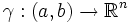Curvature notions coincide for curve in Euclidean space

Suppose$\gamma:(a,b) \to \R^n$ is a smooth curve in Euclidean space, parametrized by arc-length. Consider the following way of defining curvature for$\gamma$:
• View the image of$\gamma$ as a 1-dimensional submanifold of Euclidean space
• Use this to obtain a Levi-Civita connection on the image of$\gamma$
The curvature we get in this manner is a$(1,3)$-tensor: it takes in three tangent vectors, and outputs one tangent vector. However, since the tangent space is one-dimensional, we can view the curvature as a real number: the real number obtained when we put all three input tangent vectors as unit vectors pointing along the$\gamma$-positive direction.